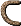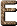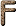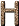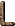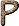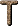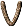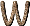q0 The cosmological deceleration parameter. QCD Quantum Chromodynamics QED Quantum Electrodynamics QEH Quantum Efficiency Hysteresis An increase in QE after exposure to light. [McL97] QSO Quasi-Stellar Object (Quasar) Q-Branch A set of lines in the spectra of molecules corresponding to changes in vibrational energy with none in rotational energy. [H76] QE Pinned The quantum efficiency of certain CCDs can be driven to their maximum by UV flooding and pinned there by immediate cooling. [McL97] Quadrant An instrument, based on a quarter of a circle, employed to measure the altitude above the horizon of astronomical bodies. Eventually replaced by the sextant. [F88] Quadrature (a) Elongation of a planet when it makes a 90° angle with the Sun as seen from Earth. (b) A configuration in which two celestial bodies have apparent longitudes (see Longitude, Celestial) that differ by 90° as viewed from a third body. Quadratures are usually tabulated with respect to the Sun as viewed from the center of the Earth. [S92] (c) A means of combining errors or noise by summing the "square" of the values and then taking the "square root". [McL97] (d) A calculation involving a definite integral. [H76] Quadrupole When referred to a system containing charges, a quadrupole is equivalent to the presence of two equal dipoles parallel to each other, but with their corresponding charges reversed; or more generally, that component of the charge distribution which has axial or triaxial symmetry. Similarly, when referred to mass distributions, it arises from unequal components of the moment-of-inertia tensor along three principal directions. [H76] Quanta (a) Fundamental units of energy. [F88] (b) Light can carry energy only in specific amounts, proportional to the frequency, as though it came in packets. The term quanta was given to these discrete packets of electromagnetic energy by Max Planck. [McL97] (c) The smallest physical units into which something can be partitioned, according to the laws of quantum mechanics. For instance, photons are the quanta of the electromagnetic field. [G99] (d) Each particle is surrounded by a field for each of the kinds of charges it carries, such as an electromagnetic field if it has electric charge. In the quantum theory, the field is described as made up of particles that are the quanta of the field. More loosely, the smallest amount of something that can exist. [K2000] Quantization The restriction of various quantities to certain discrete values; or, more generally, to deriving the quantum-mechanical laws of a system from its corresponding classical laws. [H76] Quantum A discrete quantity of energy hv associated with a wave of frequency v. It is the smallest amount of energy that can be absorbed or radiated by matter at that frequency. [H76] Quantum Chromodynamics QCD(a) The quantum field theory describing the interactions of quarks through the strong "color" field (whose quanta are gluons). QCD is a gauge theory with the non-Abelian gauge symmetry group SU(3)C. [CD99] (b) This is the accepted theory of the forces that bind quarks together to form protons, neutrons, and other strongly interacting particles. see Yang-Mills Theories [G97] (c) The quantum theory of the strong nuclear force, which it envisions as being conveyed by quanta called gluons. The name derives from the assignment of a quantum number called color to designate how quarks function in response to the strong force. [F88] (d) Relativistic quantum field theory of the strong force and quarks, incorporating special relativity. [G99] (e) The modern theory of the strong forces between quarks, and hence of the forces between hadrons. It is a generalisation of quantum electrodynamics, with color charge replacing electric charge and gluons replacing photons. [D89] Quantum Cosmology (a) The study of the Planck era. [c97] (b) The subfield of cosmology that deals with the niverse during its first 10-43 seconds, when quantum mechanical effects and gravity were both extremely important. [LB90] Quantum Defect The principal quantum number responsible for a spectral series, minus the Rydberg denominator for any actual spectral term of the series. (also called Rydberg correction) [H76] Quantum Determinism Property of quantum mechanics that knowledge of the quantum state of a system at one moment completely determines its quantum state at future and past moments. Knowledge of the quantum state, however, determines only the probability that one or another future will actually ensue. [G99] Quantum Efficiency QE (a) The efficiency of a counter in detecting photons; the probability that a photon will liberate an electron and thus be detected. [H76] (b) The ratio of the number of photoelectrons released for each incident photon of light absorbed by a detector. This ratio cannot exceed unity. [McL97] (c) The fraction of the incoming photon stream that is converted into signal. [R04] Quantum Efficiency Hysteresis QEH An increase in QE (quantum efficiency) after exposure to light. [McL97] Quantum Electrodynamics QED (a)The quantum field theory describing the interactions between electrically charged particles through the electro-magnetic field (whose quantum is the photon). QED is a gauge theory with the Abelian gauge symmetry group U(1). [CD99] (b) The theory of photons and electrons (or other electrically charged particles) and their interactions. It is called `quantum' when the electromagnetic radiation (i.e. light etc.) is being treated by quantum theory, so that its discrete photon nature is important. [D89] (c) The quantum theory of the electromagnetic force, which it envisions as being carried by quanta called photons. [F88] (d) This is the accepted theory of electromagnetic interactions, including all the effects of relativity and quantum theory. The photon acts as the carrier of the electromagnetic force. [G97] (e) Relativistic quantum field theory of the electromagnetic force and electrons, incorporating special relativity. [G99] Quantum Electronics this is the name used for those parts of quantum optics which have practical device applications.[D89] Quantum Field A distribution of energy that is constantly creating and destroying particles, according to the probabilities of quantum mechanics, and transmitting the forces of nature. see Field Theory; Quantum Mechanics [LB90] Quantum Field Theory (a) The relativistically invariant version of quantum mechanics. [H76] (b) The theory used to describe the physics of elementary particles. According to this theory, quantum fields are the ultimate reality and particles are merely the localized quanta of these fields. [CD99] (c) The theory that describes the quantum effects of a classical system of fields defined on space-time and satisfying various partial differential equations. [D89] (d) When interactions among particles are described as transmitted via the exchange of bosons, the methods of quantum field theory are used. [K2000] Quantum Fluctuations (a) The spontaneous fluctuation of energy in a volume of space. A consequence of the Heisenberg uncertainty principle. [c97] (b) Turbulent behavior of a system on microscopic scales due to the uncertainty principle. [G99] (c) Continuous variations in the properties of a physical system, caused by the probabilistic character of nature as dictated by quantum mechanics. For example, the number of photons in a box with perfectly reflecting walls is constantly varying because of quantum fluctuations. Quantum fluctuations can cause particles to appear and disappear. Some theories hold that the entire Universe was created out of nothing, in a quantum fluctuation. [LB90] Quantum Genesis (a) Hypothesis that the origin of the Universe may be understood in terms of a quantum chance. [F88] (b) A quantum theory of gravity in which gravitons transmit the force between particles, rather than the curvature of the spacetime continuum, as in the general theory of relativity. [c97] Quantum Geometry Modification of Riemannian geometry required to describe accurately the physics of space on ultramicroscopic scales, where quantum effects become important. [G99] Quantum Gravity (a) A theory of gravity that would properly include quantum mechanics. To date, there is no complete and self-consistent theory of quantum gravity, although successful quantum theories have been found for all the forces of nature except gravity. see Quantum Mechanics [LB90] (b) A general term used to describe attempts to quantize gravity. The elementary particle of the gravitational field is the graviton. [P88] (c) A theory that successfully mergers quantum mechanics and general relativity, possibly involving modifications of one or both. String theory is an example of a theory of quantum gravity. [G99] Quantum Hall Effect in a two-dimensional electron system at sufficiently low temperature and in sufficiently high magnetic field the ratio of the current to the voltage applied in a direction perpendicular to the current is very accurately a multiple (integer or fraction with small odd denominator) of e2/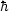, where e is the electron charge andis Planck's constant. [D89] Quantum Leap The disappearance of a subatomic particle - e.g., an electron - at one location and its simultaneous reappearance at another. The counter-intuitive weirdness of the concept results in part from the limitations of the particle metaphor in describing a phenomenon that is also in many respects a wave. [F88] Quantum Liquid a system of particles which are both sufficiently mobile and at sufficiently low temperature to display the effects of quantum-mechanical indistinguishability. Examples include the electrons in superconducting metals and the atoms in liquid helium. [D89] Quantum Mechanical Amplitude A mathematical quantity in quantum mechanics whose absolute square determines the probability of a particular process occurring. [CD99] Quantum Mechanics (a) The theory that explains the dual wave-like and particle-like behavior of matter and the probabilistic character of nature. According to quantum mechanics, it is impossible to have complete and certain information about the state of a physical system, just as a wave cannot be localized to a single point in space but spreads out over many points. This uncertainty is an intrinsic aspect of the system or particle, not a reflection of our inaccuracy of measurement. Consequently, physical systems must be described in terms of probabilities. For example, in a large collection of uranium atoms, it is possible to accurately predict what fraction of the atoms will radioactively disintegrate over the next hour, but it is impossible to predict which atoms will do so. As another example, an electron with a well-known speed cannot be localized to a small region of space but behaves as if it occupied many different places at the same time. Any physical system, such as an atom, may be viewed as existing as a combination of its possible states, each of which has a certain probability. Quantum theory has been extremely successful at explaining the behavior of nature at the subatomic level, although many of its results violate our common-sense intuition. see Copenhagen Interpretation of Quantum Mechanics; Many-Worlds Interpretation of Quantum Mechanics; Uncertainty Principle; Wave Function [LB90] (b) The theory of the interaction of matter and radiation, which rests on the original idea of Planck that radiating bodies emit energy in discrete units or quanta of radiation whose energy is proportional to the frequency of the light. [Silk90] (c) Framework of laws governing the Universe whose unfamiliar features such as uncertainty, quantum fluctuations, and wave-particle duality become most apparent on the microscopic scales of atoms and subnuclear particles. [G99] Quantum Physics Physics based upon the quantum principle, that energy is emitted not as a continuum but in discrete units. [F88] Quantum Solid A degenerate gas in which the densities are so great that the nuclei are fixed with respect to each other so that they resemble a crystalline lattice. [H76] Quantum Space Vacuum with the potential to produce virtual particles. [F88] Quantum Theory (a) A theory which seeks to explain that the action of forces is a result of the exchange of sub-atomic particles. [c97] (b) The theory used to describe physical systems which are very small, of atomic dimensions or less. A feature of the theory is that certain quantities (e.g. energy, angular momentum, light) can only exist in certain discrete amounts, called quanta. [CD99] (c) Initially, the theory developed by Planck that radiating bodies emit energy not in a continuous stream, but in discrete units called quanta, the energy of which is directly proportional to the frequency. Now, all aspects of quantum mechanics. [H76] (d) The quantum theory provides the rules with which to calculate how matter behaves. Once scientists specify what system they want to describe and what the interactions among the particles of the system are, then the equations of the quantum theory are solved to learn the properties of the system. [K2000] Quantum Tunneling A quantum leap through a barrier. [F88] (b) A process by which a quantum system can suddenly and discontinuously make a transition from an initial configuration to a final one, even if the system does not have enough energy to classically attain the configurations between the two. [G97] (c) Feature of quantum mechanics showing that objects can pass through barriers that should be impenetrable according to Newton's classical laws of physics. [G99] Quantum Yield (In photochemistry, the number of molecules decomposed per photon absorbed. [H76] Quark (a) Fundamental particle of which protons, neutrons and electrons are now thought perhaps to be made up. There are possibly three or four types of quark. It is even possible that quarks themselves may be made up of still more fundamental particles. [A84] (b) A sub-atomic particle which is a fundamental building block of the hadrons. [c97] (c) A, spin-1/2 particle with fractional electric charge (+2/3 or 1/3). Baryons are composed of three (valence) quarks which are bound together by the strong color forces, and mesons consist of a bound quark and antiquark. Quarks come in six flavors (u, d, s, c, b, t) and three colors (r, g, b). [CD99] (d) A particle that is acted upon by the strong force. Quarks exist in six varieties (up, down, charm, strange, top, bottom) and three "colors" (red, green, blue). [G99] (e) One of the fundamental, indestructible particles of nature, out of which many other subatomic particles are made. The neutron, for example, is built of three quarks. Five types of quarks have been discovered, and it is believed that a sixth also exists. Quarks interact mainly via the strong nuclear force and the electromagnetic force. [LB90] (f) The hypothetical constituent of the elementary particles that interacts via glue forces. Originally only three quarks were hypothesized; today it appears that six are required. For a variety of theoretical reasons, free quarks can never be seen. [P88] (g) Fundamental particles from which all hadrons are made. According to the theory of quantum chromodynamics, protons, neutrons, and their higher-energy cousins are composed of trios of quarks, while the mesons are each made of one quark and one antiquark. Held together by the strong nuclear force, quarks are not found in isolation in nature today. see Asymptotic Freedom [F88] (h) Fundamental particle of all hadrons. There are six known varieties (or flavors') of quarks, and they combine in twos or threes to make up particles such as protons, neutrons and mesons. [D89] (i) A fundamental particle. Quarks are very much like electrons, but they also carry strong charge and thus have another interaction, one that can bind them into protons and neutrons. There are six quarks, called up (u), down (d), charmed (c), strange (s), top (t), and bottom (b).[K2000] Quark-Hadron Phase Transition A phase transition in the early Universe when freely roaming quarks combined to form neutrons, protons, and other strongly interacting particles called hadrons. see Neutron; Phase Transition; Quark [LB90] Quark Jet Because quarks must end up in hadrons, quarks that are produced in collisions actually appear in detectors as a narrow jet of hadrons, mostly pions. see also Gluon Jet [K2000] Quasar QSO (a) The brightest objects in the Universe, quasars can generate over a trillion times as much light as the Sun from a region little larger than the Solar System. Most are extremely distant, which means that they existed long ago. [C95] (b) An intensely bright extragalactic object which superficially resembles a star. Most exist at very high redshifts and are therefore thought to be the nuclei of active galaxies. [c97] (c) Compact-looking objects, often radio sources, with emission lines in their spectrum which are displaced by very large amounts towards the red. These redshifts correspond to velocities which are a large fraction of the speed of light, and hence these objects are believed to lie at great distances. [McL97] (d) Extremely distant and luminous astronomical objects that are much smaller than a galaxy and much more luminous. Quasars may be the central regions of certain very energetic galaxies at an early stage of their evolution. It is believed that the power of a quasar derives from a massive black hole at its center. [LB90]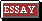An object with a dominant starlike (i.e., diameter less than 1") component, with an emission line spectrum showing a large redshift - up to z = 3.53 (0.91c) for OQ 172. (The largest redshift known for a normal radio galaxy is z = 0.637 for 3C 123.) Many have multiple absorption redshifts; a few have multiple emission redshifts. (Bahcall system: class I, zabszem; class II, zabs significantly less than zem.) The light of most if not all quasars is variable over time intervals between a few days and several years, so their diameters must not be much larger than the diameter of the Solar System; yet they are the intrinsically brightest objects known (for 3C 273 (z = 0.158), Mv = -27.5 if its redshift is cosmological). The energy output of a typical quasar at "cosmological" distance is of the order of 1047 ergs per second - which would require a mass of 1010 M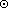if it derives its energy solely from nuclear fusion. (Energy requirement under the "local" hypothesis is on the order of 1042 ergs per second.) The basic problem of quasars is that they emit too much radiation in too short a time from too small an area. [H76] (e) An extreme form of active galactic nucleus in which the luminosity of the nucleus far exceeds the luminosity of the underlying galaxy. As a result, these objects have a stellar appearance on photographic plates. The first members of this class were discovered through the optical identification of extragalactic radio sources and hence the origin of the name `quasi-stellar radio source' abbreviated to quasar. The quasars are the most luminous objects known in the Universe. [D89] Quasi-Stellar Radio Source QSRS A quasar with detectable radio emission. [H76] Quiet Sun The Sun when the 11-year cycle of activity is at a minimum. [H76]